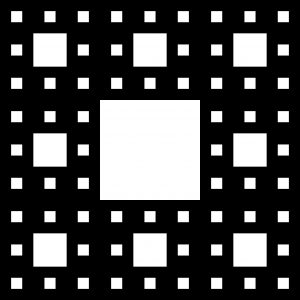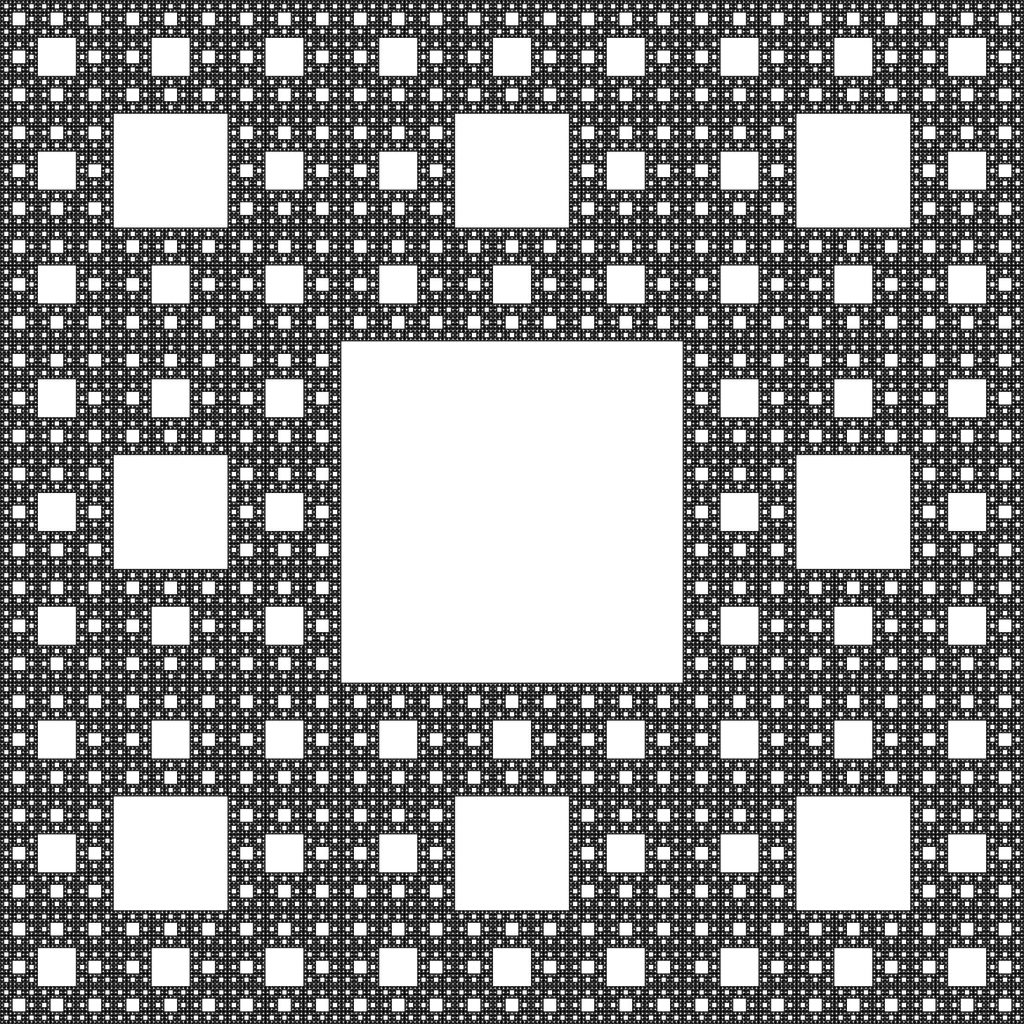# Python | Sierpinski Carpet

The Sierpinski Carpet is a plane fractal curve i.e. a curve that is homeomorphic to a subspace of plane. It was first described by Waclaw Sierpinski in 1916. In these type of fractals, a shape is divided into a smaller copy of itself, removing some of the new copies and leaving the remaining copies in specific order to form new shapes of fractals.

How is it constructed?
The Sierpinski Carpet starts with a square. This square is divided into nine equal parts. The centremost smaller square is removed from the original larger square. The remaining square pieces are then again divided into nine equal parts and the centermost square from each square is removed. On repeating this process, a beautiful pattern of Sierpinski Carpet is observed.We divide it into 9 equal pieces and remove the center square.On further repeating the process, it results in something like this.We can visualize this phenomenon in details in this video.

Let us see what its code looks like :

 `# importing necessary modules ` `import` `numpy as np ` `from` `PIL ``import` `Image ` ` `  `# total number of times the process will be repeated ` `total ``=` `7` ` `  `# size of the image ` `size ``=` `3``*``*``total ` ` `  `# creating an image ` `square ``=` `np.empty([size, size, ``3``], dtype ``=` `np.uint8) ` `color ``=` `np.array([``255``, ``255``, ``255``], dtype ``=` `np.uint8) ` ` `  `# filling it black ` `square.fill(``0``) ` ` `  `for` `i ``in` `range``(``0``, total ``+` `1``): ` `    ``stepdown ``=` `3``*``*``(total ``-` `i) ` `    ``for` `x ``in` `range``(``0``, ``3``*``*``i): ` `         `  `        ``# checking for the centremost square ` `        ``if` `x ``%` `3` `=``=` `1``: ` `            ``for` `y ``in` `range``(``0``, ``3``*``*``i): ` `                ``if` `y ``%` `3` `=``=` `1``: ` `                     `  `                    ``# changing its color ` `                    ``square[y ``*` `stepdown:(y ``+` `1``)``*``stepdown, x ``*` `stepdown:(x ``+` `1``)``*``stepdown] ``=` `color ` ` `  `# saving the image produced ` `save_file ``=` `"sierpinski.jpg"` `Image.fromarray(square).save(save_file) ` ` `  `# displaying it in console ` `i ``=` `Image.``open``(``"sierpinski.jpg"``) ` `i.show() `

Output :This is the Sierpinski Carpet after 7 repetitions. You can get its code for other languages on rosettacode.

Whether you're preparing for your first job interview or aiming to upskill in this ever-evolving tech landscape, GeeksforGeeks Courses are your key to success. We provide top-quality content at affordable prices, all geared towards accelerating your growth in a time-bound manner. Join the millions we've already empowered, and we're here to do the same for you. Don't miss out - check it out now!